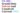# CS 502 – Grand Quiz Solved and Unsolved Current 2021

0
113### CS 502 – Grand Quiz Solved and Unsolved Current 2021

Dynamic programming strategy is useful when sub-problems are independent.

1. True
2. False

As per algorithm of Dynamic programing .we need to store the result(s) of

1. First sub-problem only
2. Best solution only
3. Intermediate sub-problem
4. Final solution only

In the following code the statement “cout<<I,”executes………………..times.

For(int i = 1 ; i<=n ;i++)

Cout<<I;

1. N time
2. N+5 time
3. Infinite times
4. Zero time

Brute-force algorithm for 2D-Maxima is operated by comparing_________pairs of points.

1. Two
2. Some
3. Most
4. All

Quick sort is ____ based on Divide and Conquer strategy.

1. Not
2. Always
3. Sometimes
4. Occasionally

Memorization is a part of Dynamic Programming strategy.

1. True
2. False

While designing brute force algorithm _______ is not given much importance.

1. Input
2. Output
3. Processing
4. Efficiency

We can improve the performance of Quick sort if we can be able to __________

1. Skip input elements somehow
2. Select two or more pivots
3. Skip any sub-array completely
4. Eliminate recursive calls

CS 502 – Grand Quiz Solved and Unsolved Current 2021

Asymptotic growth of plan –sweep algorithm for 2-D maxima problem is _____.

1. @(n)
2. @(logn)
3. @(nlogn)
4. @(n^2)

Merge sort is based on___________

1. Brute-force
2. Plan-sweep
3. Axis-sweep
4. Divide and Conquer

In Heap Sort algorithm .we build _________for ascending sort.

1. Max heap
2. Min heap

The worst case running time of Quick sort algorithm

3. Is always Exponential
4. Is linear

If there are @(n2) entries in edit distance matrix then each entry E (I ,j) takes ———–time to compute.

1. @ (n)
2. @ (n2)
3. @ (1)
4. @ (n log n)

In order to say anything meaningful about our algorithms , it will be important for us to settle on a

1. Java Program
2. C++ program
3. Pseudo program
4. Mathematical model of computation

Dynamic programming approach is usually useful in solving optimization problems.

1. True
2. False

_______ items are not allowed in the 0/1 knapsack

1. Lighter
2. Fractional
3. Whole
4. Weighty

In merge sort algorithm ,we split the array around the _________index q.

1. Entring
2. Mid
3. Exiting
4. Summing

For comparison-based sorting algorithms ,it is ____ possible to sort more efficiently than Omega nlog time.

1. Always
2. Not
3. Sometimes
4. Sometimes not

Algorithm is a sequence of computational step that ____ the input into output

1. Merge
2. Assign
3. Transform
4. Integrate

The asymptotic growth of n(n+1)/2 is:

1. O(n2)
2. O(n)
3. O(n+2)
4. O (n log n)

Rank of an element can be define as ___________

1. One minus the number of element that are smaller
2. Two plus the number of elements that are greater
3. One plus the number of elements that are smaller
4. Two minus the number of elements that are smaller

In the following code the statement “cout<<j;execute ………………times.

For (j = 1; j<=5 ;j = j+2)

Count<<j;

1. 5 times
2. 2 times
3. 3 times
4. 0 times

Insertion sort is an in-pace sorting algorithm

1. True
2. False

Which one is not passed as parameter in Quick sort algorithm?

1. End of the array
2. Middle of the array
3. Array (containing input elements)
4. Start of the array

The running time of Quick sort algorithm____________

1. Is impossible to compute
2. Has nothing to do with pivot selection
3. Is Random upon each execution
4. Greatly influenced by the selection of pivot